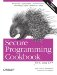# Copying a vector

Problem

You need to copy the contents of one vector into another.

Solution

There are a couple of ways to do this. You can use a copy constructor when you create a vector, or you can use the assign member function. Example 6-3 shows how to do both.

Example 6-3. Copying vector contents

```#include
#include
#include
#include

using namespace std;

// Util function for printing vector contents
template
void vecPrint (const vector& vec) {
cout << "{";
for (typename vector::const_iterator p = vec.begin( );
p != vec.end( ); ++p) {
cout << "{" << *p << "} ";
}
cout << "}" << endl;
}

int main( ) {

vector vec(5);
string foo[] = {"My", "way", "or", "the", "highway"};

vec = "Today";
vec = "is";
vec = "a";
vec = "new";
vec = "day";

vector vec2(vec);
vecPrint(vec2);

vec.at(0) = "Tomorrow";

vec2.assign(vec.begin( ), vec.end( )); // Copy each element over
vecPrint(vec2); // with assign

vec2.assign(&foo, &foo); // Assign works for anything that
vecPrint(vec2); // behaves like an iterator

vector::iterator p;

p = find(vec.begin( ), vec.end( ), "new");

vec2.assign(vec.begin( ), p); // Copy a subset of the full range
vecPrint(vec2); // of vec
}```

Discussion

Copying a vector is easy; there are two ways to do it. You can copy construct one vector from another, just like any other object, or you can use the assign member function. There is little to say about the copy constructor; just pass in the vector you want it to clone, and you're done.

`vector vec2(vec);`

In this case, vec2 will contain the same number of elements that are in vec, and each one of those elements will be a copy of its corresponding index in vec. Each element is copied with string's copy constructor. Since this is construction, vec2's buffer is sized at least large enough to hold everything in vec.

assign works similarly, except that there is some additional work that goes on behind the scenes, since now you are dealing with a target vector that may already have data in it. First, the objects that are in the way, so to speak, must be destroyed. assign first calls the destructor for each of the objects that vec2 already contains. Once they are gone, it checks vec2's buffer size to ensure it is big enough to hold what it is about to receive from vec. If not, assign resizes the buffer to accommodate the new data. Finally, it copies each element over.

Additionally, you can use assign for copying a subset of a sequence. For example, if you just want to assign a subset of the elements in vec, just specify the range you want to pull when calling assign:

```vector::iterator p;
p = std::find(vec.begin( ), vec.end( ), "new");
vec2.assign(vec.begin( ), p);
vecPrint(vec2);```

In this case, assign will copy everything up to, but not including, p. This is because, as is the convention in all standard library containers and algorithms, assign(first, last) copies the element pointed to by first up to, but not including, the element pointed to by last. Such a range, that includes the first element but not the last element, is often denoted as [first, last).

Use assign or the copy constructor instead of looping yourself. That is, don't copy each element by looping through vec and pushing each element on the back of vec2. This requires more (redundant) code on your part, and disallows any optimizations the implementer of your standard library may have used when writing assign or the copy constructor.Secure Programming Cookbook for C and C++: Recipes for Cryptography, Authentication, Input Validation & More
ISBN: 0596003943
EAN: 2147483647
Year: 2006
Pages: 241